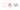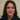# What is ES6 Set object in Javascript and how to use it

In this article we will describe what a set object is in Javascript and how to use it in our code. It is very compatible with other  ES6 features by the way.

## Set object

As MDN describes the set object is a collection of only unique values of any type. Primitive values or object references. And it is iterable just like an array:

``new Set([<em>iterable</em>]);``

And this is how we initialise it in the code:

``````const newSet1 = new Set();
const newSet2 = new Set(null);
const newSet3 = new Set([1, 2, 3, 4, 5]);``````

Logically the add method adds new entries to the set collection and if ever a duplication happens based on strict equality of set it will be discarded. As mentioned above set only contains unique values. This is an example of creating a set collection and iterate through it using the forEach loop:

``````const newSet = new Set();
newSet.forEach(el => {
console.log(el);
// expected output: C
// expected output: 1
// expected output: H
});``````

Moreover we could check if a value is in our set object using the has method:

``````const newSet = new Set(["A", 2, "B", 4, "C"]);
console.log(newSet.has("A"));
// expected output: true
console.log(newSet.has(4));
// expected output: true
console.log(newSet.has(5));
// expected output: false``````

As a result it returns a boolean value of true if that value exists in the set object and false if it doesn’t.

Delete or clear an element

As another functionality we could also delete a value from the set collection. And even check the size of a set:

``````const newSet = new Set(["A", 2, "B", 4, "C"]);
newSet.delete("C");
// expected output: true
newSet.delete("C");
// expected output: false
newSet.size
// expected output: 4

newSet.clear();
// expected output: undefined
newSet.size
// expected output: 0``````

### Iterating over the set collection

As an interesting part when we need to iterate over set object,  just like arrays we could use all relevant methods in this case. Take a look at this MDN example with its comments:

``````var mySet = new Set();

mySet.add(1); // Set [ 1 ]
mySet.add(5); // Set [ 1, 5 ]
mySet.add(5); // Set [ 1, 5 ]
mySet.add('some text'); // Set [ 1, 5, 'some text' ]
var o = {a: 1, b: 2};

mySet.add({a: 1, b: 2}); // o is referencing a different object so this is okay<br/><br/>// iterate over items in set
// logs the items in the order: 1, "some text", {"a": 1, "b": 2}, {"a": 1, "b": 2}
for (let item of mySet) console.log(item);

// logs all items in the order: 1, "some text", {"a": 1, "b": 2}, {"a": 1, "b": 2}
for (let item of mySet.keys()) console.log(item);

// logs items in the order: 1, "some text", {"a": 1, "b": 2}, {"a": 1, "b": 2}
for (let item of mySet.values()) console.log(item);

// logs the items in the order: 1, "some text", {"a": 1, "b": 2}, {"a": 1, "b": 2}
//(key and value are the same here)
for (let [key, value] of mySet.entries()) console.log(key);

// convert Set object to an Array object, with Array.from
var myArr = Array.from(mySet); // [1, "some text", {"a": 1, "b": 2}, {"a": 1, "b": 2}]

// the following will also work if run in an HTML document
mySet.has(document.querySelector('body')); // true

// converting between Set and Array
mySet2 = new Set([1, 2, 3, 4]);
mySet2.size; // 4
[...mySet2]; // [1, 2, 3, 4]

// intersect can be simulated via
var intersection = new Set([...set1].filter(x => set2.has(x)));

// difference can be simulated via
var difference = new Set([...set1].filter(x => !set2.has(x)));

// Iterate set entries with forEach
mySet.forEach(function(value) {
console.log(value);
});

// 1
// 2
// 3
// 4``````

#### What is the example about

Fistly creating set in the first few lines it is using different kind of for loop methods (for …of ) to log set object into the console.

Afterwards it converts it into an array and using spread operator creates copies of the old set and filters through hem. We can even add document.body to set collection. And finally print it out using forEach loop.

Technically the set object is really useful if we need to keep a collection of unique values of different objects that is an iterable array and can be manipulated as long as the values stay unique. Thanks for reading.